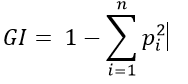Classification

Decision Tree

Machine Learning

Regression

January 8, 2021

# What is Gini Impurity?

According to Wikipedia, ‘Gini impurity is a measure of how often a randomly chosen element from the set would be incorrectly labelled if it was randomly labelled according to the distribution of labels in the subset.’

Like entropy, Gini Impurity too is a measure of randomness of data. Randomness signifies the heterogeneity of labels. Decision trees split the data in manner that leads to decrease in Gini Impurity. Thus Decision Trees aim to divide the data with heterogenous labels into subsets/sub-regions of data with homogenous labels. Thus with each division level of homogeneity increases and Gini Impurity decreases. In fact Gini Impurity is the cost function that decision trees employ as basis of splitting the data, if the the split leads to decrease in Gini Impurity then it’s carried out else not.

For a dataset with n classes, the formula for Gini Impurity would be:Where p is the probability of occurrence of each class.

by : Monis Khan

Quick Summary:

According to Wikipedia, ‘Gini impurity is a measure of how often a randomly chosen element from the set would be incorrectly labelled if it was randomly labelled according to the distribution of labels in the subset.’ Like entropy, Gini Impurity too is a measure of randomness of data. Randomness signifies the heterogeneity of labels. Decision […]